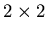MAT331
Exercise 3: Simple Cryptography

1.
Decode the following phrase, which was encoded using a affine encoding cipher on a 27-letter alphabet (the letters a-z and a blank).
Recall that a Vignère cipher can be interpreted as a Caesar-like cipher on n-vectors, where n is the length of the key phrase. Can any affine encipherment on digraphs (two-character codes) be interpreted as a an affine matrix encipherment on 2-vectors? That is, suppose I encode a message by affine enciphering on digraphs. Can I get the same crypttext from the same plaintext using an affine matrix enciphering (using amatrix) on 2-vectors? If your answer is yes, prove it. If no, give a counter-example that cannot be so interpreted.